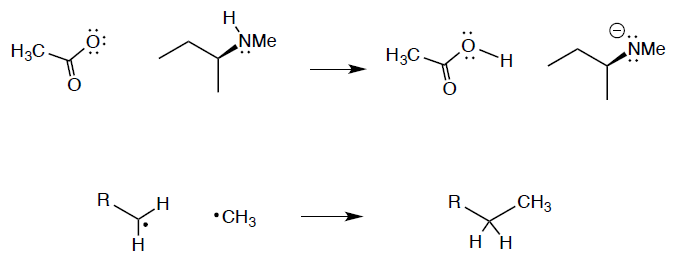Problem: For each of the following one step transformations show the movement of electrons by using the standard curved arrow notation. Show all formal charges for each structure on the left and right of the reaction arrows.

FREE Expert Solution
96% (101 ratings)
Problem Details

For each of the following one step transformations show the movement of electrons by using the standard curved arrow notation. Show all formal charges for each structure on the left and right of the reaction arrows.What scientific concept do you need to know in order to solve this problem?

Our tutors have indicated that to solve this problem you will need to apply the Reaction Mechanism concept. You can view video lessons to learn Reaction Mechanism Or if you need more Reaction Mechanism practice, you can also practice Reaction Mechanism practice problems .

What is the difficulty of this problem?

Our tutors rated the difficulty of For each of the following one step transformations show the ... as low difficulty.

How long does this problem take to solve?

Our expert Organic tutor, Nicolle took 3 minutes to solve this problem. You can follow their steps in the video explanation above.

What professor is this problem relevant for?

Based on our data, we think this problem is relevant for Professor Huang's class at Sacramento City College.